# 10.2 Compounds of chlorine  (Page 2/2)

 Page 2 / 2## Oxides of chlorine

Chlorine forms a series of oxides ( [link] ) in which the chlorine has the formal oxidation states +1, +4, +6, and +7. The physical properties of the oxides are summarized in [link] . While, the oxides of chlorine are not very stable (in fact several are shock sensitive and are prone to explode) the conjugate oxyacids are stable.

 Compound Mp (°C) Bp (°C) Cl 2 O -116 4 ClO 2 -5.9 10 Cl 2 O 4 -117 44.5 Cl 2 O 6 3.5 unstable Cl 2 O 7 -91.5 82

Dichlorine monoxide (Cl 2 O, [link] a) is a yellowish-red gas that is prepared by the reaction of chlorine with mercury oxide, [link] , or with a solution of chlorine in CCl 4 .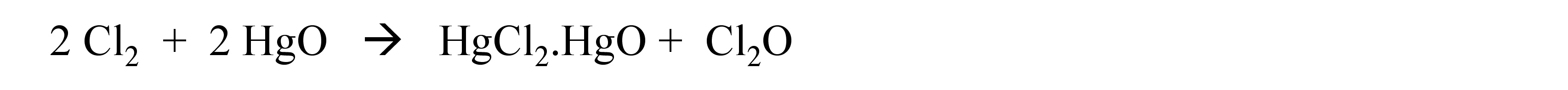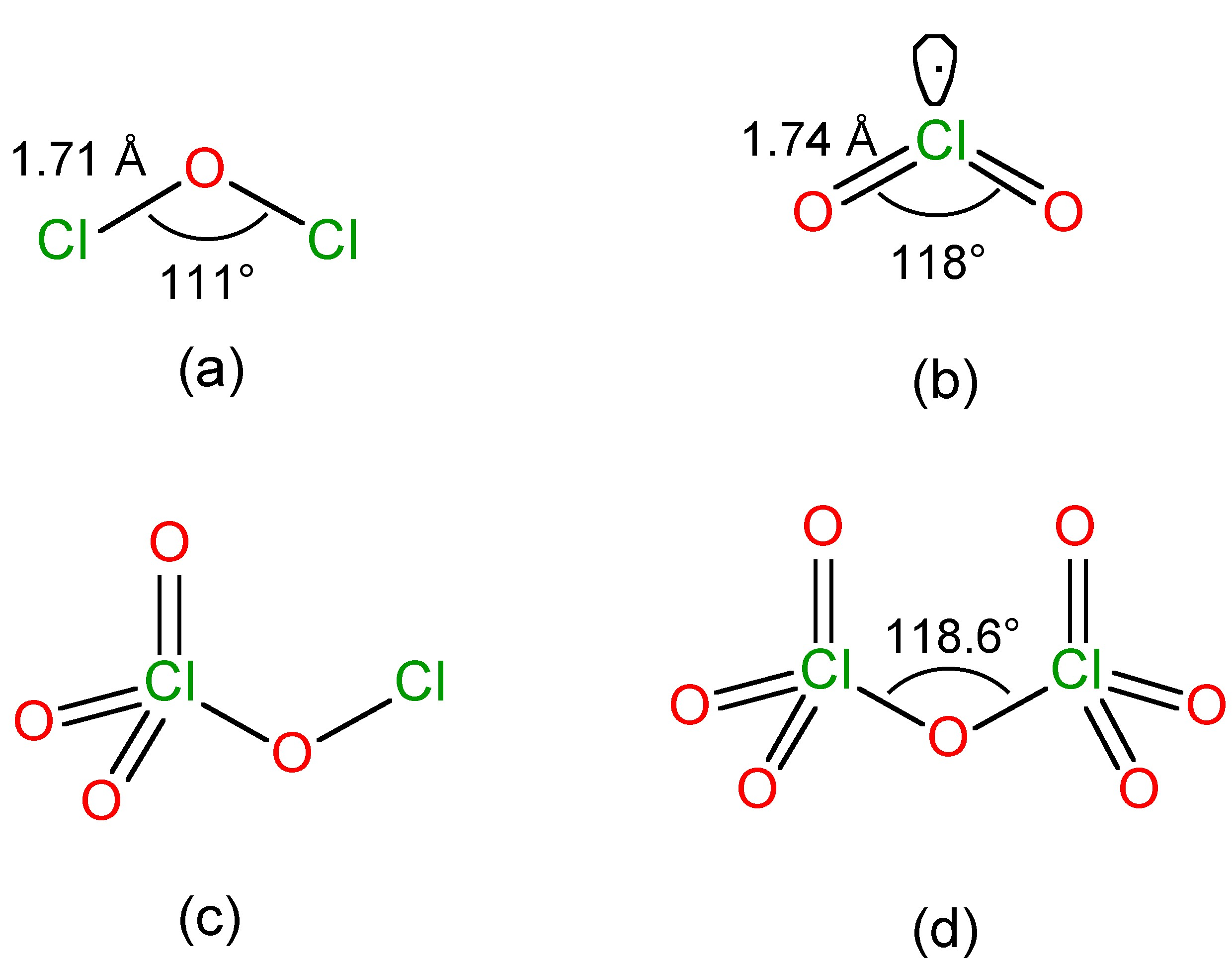The structure of (a) Cl 2 O, (b) ClO 2 , (c) Cl 2 O 4 , and (d) Cl 2 O 7 .

When heated or subject to a spark, Cl 2 O explodes to Cl 2 and O 2 . Dichlorine monoxide reacts with water to form an orange-yellow solution of hypochlorous acid, [link] .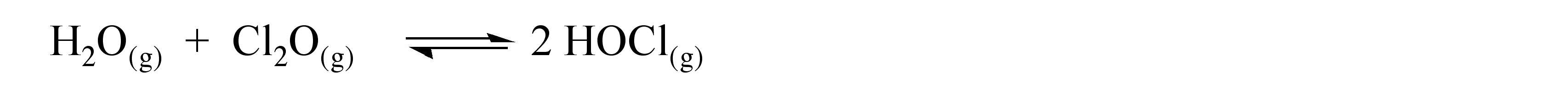Chlorine dioxide (ClO 2 ) is a yellowish gas at room temperature and is commonly used in industry as an oxidizing agent. The best synthesis of ClO 2 involves the reduction of potassium chlorate (KClO 3 ) by oxalic acid at 90 °C, since the CO 2 formed acts as a diluent for the highly explosive ClO 2 . On an industrial scale ClO 2 is made by the exothermic reaction of sodium chlorate with SO 2 in sulfuric acid, [link] . The photolysis of ClO 2 yields a dark brown solid with the formula Cl 2 O 3 ; however, its facile explosive decomposition precludes study.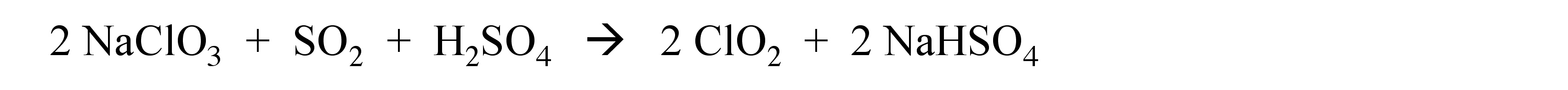The structure of ClO 2 ( [link] b) is equivalent to SO 2 with one extra electron, resulting in a paramagnetic unpaired electron species. Unusually, despite the unpaired electron configuration, ClO 2 shows no tendency to dimerize. This is unlike the analogous NO 2 molecule.

Dichlorine tetraoxide (Cl 2 O 4 ) is commonly called chlorine perchlorate as a consequence of its structure ( [link] c). Dichlorine hexaoxide (Cl 2 O 6 ) is an unstable red oil that has the ionic structure in the solid state: [ClO 2 ] + [ClO 4 ] - .

Dichlorine heptoxide (Cl 2 O 7 ) is a relatively stable oil, that is prepared by the dehydration of perchloric acid at -10 °C, [link] , followed by vacuum distillation. The structure of Cl 2 O 7 ( [link] d) has been determined by gas phase electron diffraction.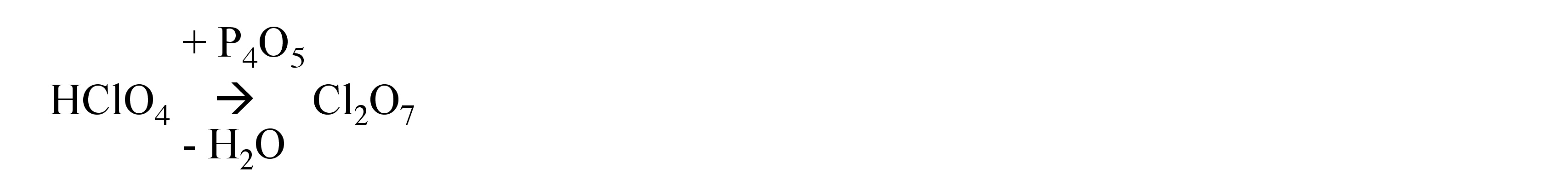The reaction of Cl 2 O 7 with alcohols and amines yields alkyl perchlorates (ROClO 3 ) and amine perchlorates (R 2 NClO 3 ), respectively.

## Fluorides of chlorine

Given the isolobal relationship between the halogens it is not surprising that the mixed dihalogens can be prepared, e.g., ClF, ICl, and BrCl. Chlorine fluoride is a highly reactive gas (Bp = -100.1 °C) that is a powerful fluorinating agent, and is prepared by the oxidation of chlorine by chlorine trifluoride, [link] .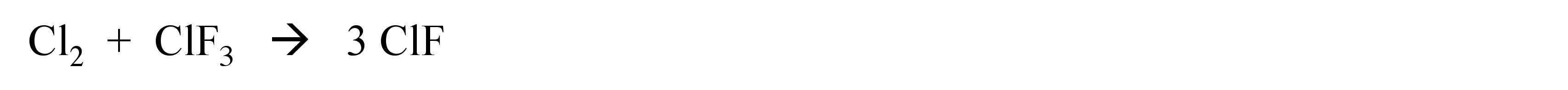The higher electronegativity of fluorine as compared to chlorine ( [link] ), and the ability of chlorine to form more than one bond, means that higher fluorides of chlorine are also known, i.e., ClF 3 and ClF 5 . Chlorine trifluoride (CF 3 , Bp = 11.75 °C) is a useful fluorinating agent, that is prepared by the high temperature reaction of elemental chlorine and fluorine, is a useful fluorinating age. The gaseous pentafluoride (ClF 5 , Bp = -31.1 °C) is prepared by the reaction of potassium chloride with fluorine, [link] .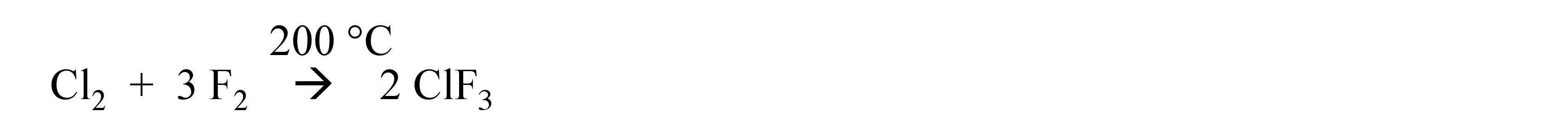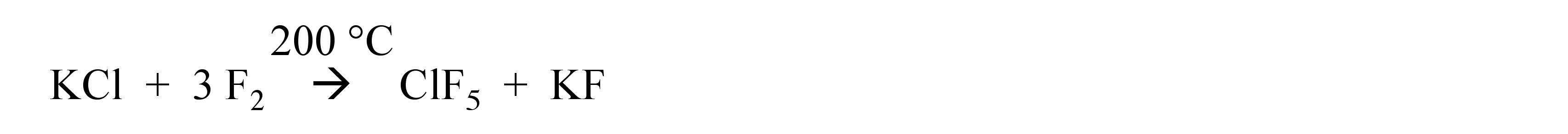The structure of ClF 3 is T-shaped with two lone pairs on chlorine ( [link] a), while that of ClF 5 is square pyramidal with a single lone pair on chlorine ( [link] b).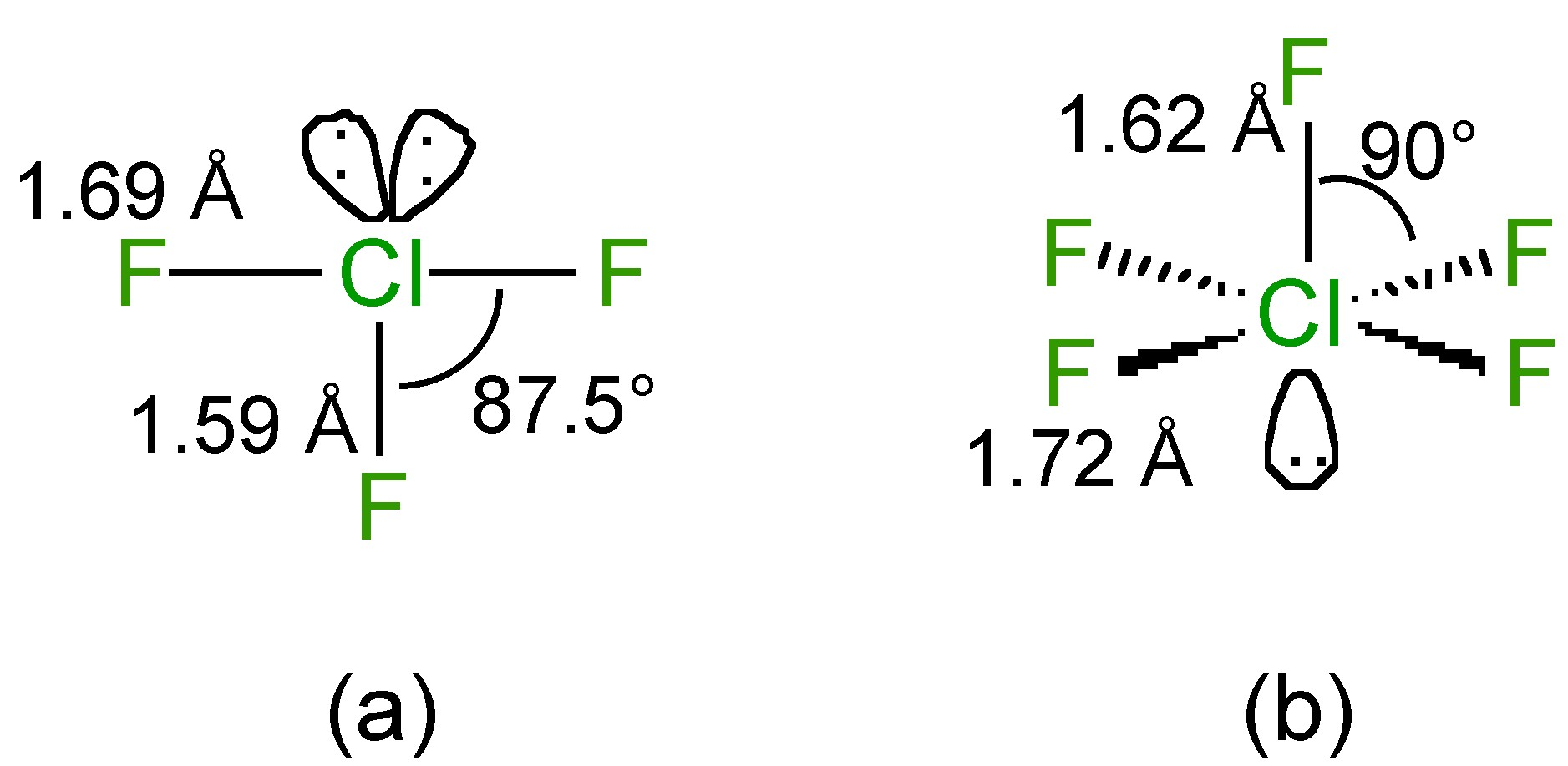The structures of (a) ClF 3 and (b) ClF 5 .

In general the halogen fluorides are very reactive; explosive reactions occur with organic compounds. They are all powerful fluorinating agents when diluted with nitrogen, and the order of reactivity follows: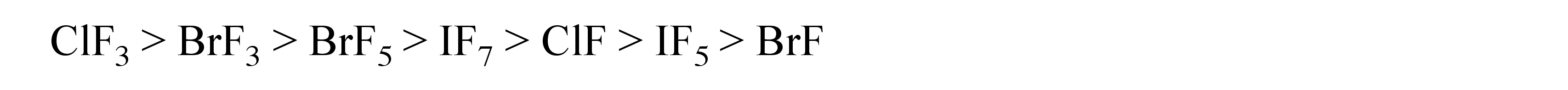Like most halogen fluorides, ClF, ClF 3 and ClF 5 all react with strong bases (e.g., alkali metal fluorides) to form anions, [link] and [link] , and strong acids (e.g., AsF 5 and SbF 5 ) to form cations, [link] , [link] , and [link] .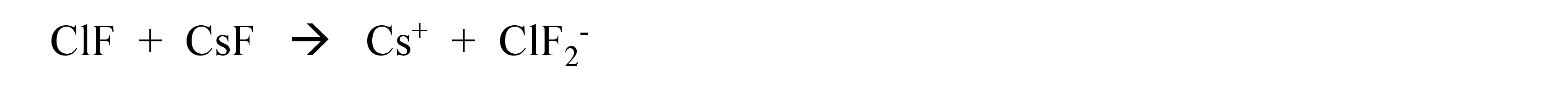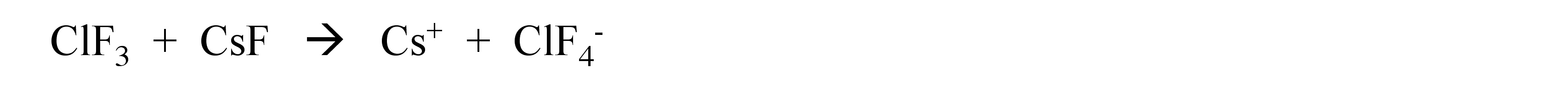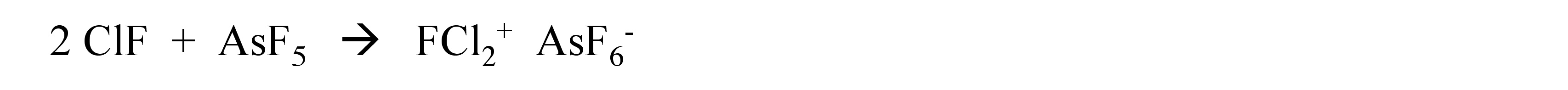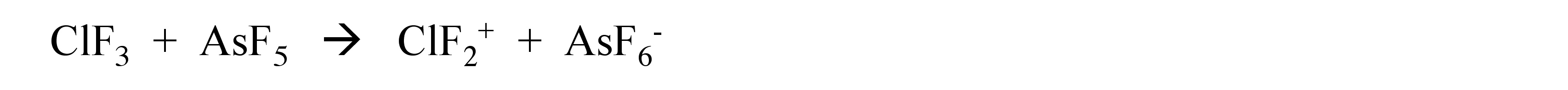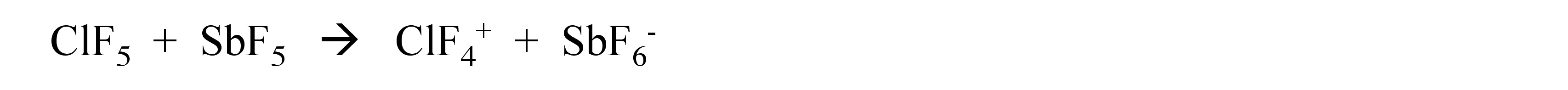what is math number
x-2y+3z=-3 2x-y+z=7 -x+3y-z=6
Need help solving this problem (2/7)^-2
x+2y-z=7
Sidiki
what is the coefficient of -4×
-1
Shedrak
the operation * is x * y =x + y/ 1+(x × y) show if the operation is commutative if x × y is not equal to -1
An investment account was opened with an initial deposit of \$9,600 and earns 7.4% interest, compounded continuously. How much will the account be worth after 15 years?
lim x to infinity e^1-e^-1/log(1+x)
given eccentricity and a point find the equiation
12, 17, 22.... 25th term
12, 17, 22.... 25th term
Akash
College algebra is really hard?
Absolutely, for me. My problems with math started in First grade...involving a nun Sister Anastasia, bad vision, talking & getting expelled from Catholic school. When it comes to math I just can't focus and all I can hear is our family silverware banging and clanging on the pink Formica table.
Carole
I'm 13 and I understand it great
AJ
I am 1 year old but I can do it! 1+1=2 proof very hard for me though.
Atone
hi
Not really they are just easy concepts which can be understood if you have great basics. I am 14 I understood them easily.
Vedant
hi vedant can u help me with some assignments
Solomon
find the 15th term of the geometric sequince whose first is 18 and last term of 387
I know this work
salma
The given of f(x=x-2. then what is the value of this f(3) 5f(x+1)
hmm well what is the answer
Abhi
If f(x) = x-2 then, f(3) when 5f(x+1) 5((3-2)+1) 5(1+1) 5(2) 10
Augustine
how do they get the third part x = (32)5/4
make 5/4 into a mixed number, make that a decimal, and then multiply 32 by the decimal 5/4 turns out to be
AJ
how
Sheref
can someone help me with some logarithmic and exponential equations.
20/(×-6^2)
Salomon
okay, so you have 6 raised to the power of 2. what is that part of your answer
I don't understand what the A with approx sign and the boxed x mean
it think it's written 20/(X-6)^2 so it's 20 divided by X-6 squared
Salomon
I'm not sure why it wrote it the other way
Salomon
I got X =-6
Salomon
ok. so take the square root of both sides, now you have plus or minus the square root of 20= x-6
oops. ignore that.
so you not have an equal sign anywhere in the original equation?
hmm
Abhi
is it a question of log
Abhi
🤔.
Abhi
I rally confuse this number And equations too I need exactly help
salma
But this is not salma it's Faiza live in lousvile Ky I garbage this so I am going collage with JCTC that the of the collage thank you my friends
salma
Commplementary angles
hello
Sherica
im all ears I need to learn
Sherica
right! what he said ⤴⤴⤴
Tamia
hii
Uday
hi
salma
hi
Ayuba
Hello
opoku
hi
Ali
greetings from Iran
Ali
salut. from Algeria
Bach
hi
Nharnhar
waht is hydrating power of lithium carbonatesByByByBy Jordon HumphreysBy